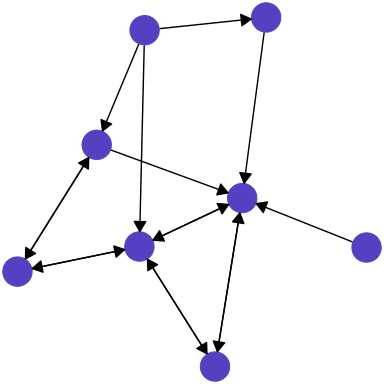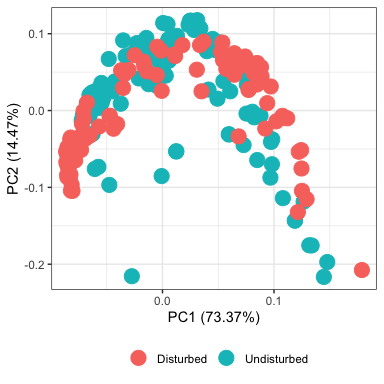Abstract

The netcom package provides tools for inferring system functioning from network data by comparing networks to each other. This is where its name comes from: network comparisons. netcom can compare networks describing any kind of empirical or theoretical system that can be stored as a matrix. Patterns in the resulting state space of inter-network distances capture system functioning, risk, and underlying mechanisms. This is particularly useful because network data are ubiquitous, reflecting a burgeoning systematics paradigm across science.

Installation

You can install the netcom package two main ways. A release version of the package can be installed from CRAN (the Comprehensive R Archive Network): https://cran.r-project.org/package=netcom.

install.packages("netcom")

Alternatively, the (usually) more recent development version can be installed from GitHub: https://github.com/langendorfr/netcom. This can be accomplished with the devtools package. We recommend new users install the other version, from CRAN, which is has less functioning but has been more reliably tested.

devtools::install_github("langendorfr/netcom")

Once installed simply load the package and you will have access to all of its functionality. Here we also load data manipulation and visualization packages necessary for the rest of this vignette.

library(netcom)     # This library
library(tibble)     # Data format
library(dplyr)      # Data processing
library(reshape2)   # Data manipulation
library(expm)       # Matrix multiplication
library(igraph)     # General puprose network library
library(ggplot2)    # Plotting using the grammar of graphics
library(ggraph)     # Graph plotting extension for ggplot2
library(ggfortify)  # Statistical plotting extension for ggplot2

This vignette covers three primary uses of netcom: simulating, comparing, and classifying networks.

Simulating Networks

Network simulation is a powerful way to study complex systems. By simulating networks according to different mechanisms (network generating recipes) you can study the kinds of systems that emerge. By comparing these networks to each other you can classify networks, and by comparing them to empirical network data you can quantify the probability that a particular mechanism is at play in a given real world system.

netcom can simulate networks according to five common mechanisms:

1. Erdos-Renyi random (ER)1
2. Preferential Attachment (PA)2
3. Duplication and Divergence (DD)3
4. Small-World (SW)4
5. Niche Model (NM)5

A future version of netcom will introduce the ability to add new mechanisms.

There are two ways to simulate a mechanism. (i) Grow a network adding nodes one at a time. Each new node can only use information about already existing nodes in determining which to interact with (share an edge). (ii) Take an already formed network and have a node re-choose which of the other nodes to interact with (share an edge). This is called rewiring. Notably, the sequence of rewired nodes need not be a unique ordered list of the nodes in the network. Nodes can be rewired multiple times or never. Growing networks imparts a chronological hierarchy, where no edge is bidirectional. This captures system evolution where novel entites are introduced, but is less able to describe systems of continously interacting variables. Rewired networks are more appropriate here, but can be biased by the starting network and the order in which nodes are rewired. Also, rewiring nodes enough times to reach an equilibrium can be computationally intensive.

Mixture Networks

Note that both ways of simulating networks (growing and rewiring) involve assigning edges to one node at a time. This allows us to combine methods and mechanisms. We can simulate a network that grows, but every so often experiences rewiring, which may characterize disturbed systems. We can also simulate networks where nodes do not all act according to the same mechanism. These kinds of systems, which we call mixture networks, may be common.6

As an example, here is a recipe for a realistically complex mixture network with a dynamic evolution:

## Start by creating a sequence of network evolutions. There are four components to this sequence that can be defined for each step in the network's evolution, or once which will be used for every step in the newtwork's evolution.
mechanism <- c(
rep("ER", 7),
rep("PA", 2),
rep("ER", 3)
)

kind <- c(
rep("grow", 7),
rep("rewire", 2),
rep("grow", 3)
)

parameter <- c(
rep(0.3, 7),
rep(2, 2),
c(0.2, 0.4, 0.3)
)

directed <- c(
rep(TRUE, 7),
rep(FALSE, 2),
c(FALSE, FALSE, TRUE)
)

## Simulate a network according to the rules of this system evolution.
network <- netcom::make_Mixture(mechanism = mechanism, kind = kind, parameter = parameter, directed = directed)

This is a system that grows randomly at first, adding seven nodes that attach to other nodes according to the Erdos-Renyi (ER) random model. Note these first seven elements of kind are “grow” signifying these are events where nodes are added to the network. The next two events are “rewire” which does not add any new nodes. Instead, an existing node replays its mechanism in the context of the currently existing network.Diagram of this mixture network’s evolution for 12 time steps.

Please note that the first two steps in the network’s evolution will always, regardless of the sequences you specify, result in two nodes that bidirectionally interact. This is because some mechanisms require an existing network. Preferential Attachment (PA) is like this. New nodes attach to existing nodes based on how many other nodes already share an edge with each existing node.

## Lastly, plot the network you've simulated.
network %>%
reshape2::melt() %>%
dplyr::filter(value == 1) %>%
ggraph::ggraph(layout = "stress") +
theme_void() +
geom_node_point(size = 10,
color = "slateblue") +
length = unit(3, "mm")),
start_cap = circle(4, "mm"),
end_cap = circle(4, "mm"))Currently a network evolution has four components, the input parameters mechanism, kind, parameter, and directed. A future version of netcom will introduce the ability to add new ones.

Comparing Networks

Aligning two networks is a common way to compare them to each other. This works by pairing nodes between the two networks, typically as a mathematical injection; ‘one-to-one’ but not necessarily ‘onto’. You can visualize this as stacking one network on top of the other. A common way to do this is to minimize the disagreement in their edges. Looking at the two networks stacked together, this is the number of places where there is only one edge, indicating that one of the networks claims there is an interaction between the two nodes connected by the edge and the other thinks those two nodes describe variables that do not interact.

Network alignment offers useful insights at both the level of the entire network and the level of individual nodes. Paired nodes suggest variables that may serve similar functional roles in their respective systems, and may even respond similarly to disturbances. This kind of information sharing is particularly helpful in systems that are difficult to study but have closely related systems that are more common, for example a species that is endangered in one country but common in another. Zooming out to the level of entire networks, network alignment offers a way to map state spaces of systemic properties, structures, and functions.

## Create two sets of 100 networks each, all with 20 nodes. One of these sets is grown
## according to the Duplication and Divergence mechanism (DD). The other is also grown in this
## manner, but with one randomly rewired node for every three nodes grown, mimicking a
## disturbed system.
num_networks <- 100

## First set of undisturbed networks.
networks_undisturbed <- list()
for (net in 1:num_networks) {
networks_undisturbed[[net]] = netcom::make_Mixture(
mechanism = rep("DD", 20),
parameter = 0.2,
directed = TRUE
)
}

## Second set of disturbed networks
networks_disturbed <- list()
for (net in 1:num_networks) {
networks_disturbed[[net]] = netcom::make_Mixture(
mechanism = rep(c("DD", "DD", "DD", "ER"), 5),
parameter = rep(c(0.2, 0.2, 0.2, 0.76), 5),
kind = rep(c("grow", "grow", "grow", "rewire"), 5),
directed = TRUE
)
}

## Pairwise compare all of the networks to each other
networks <- c(networks_undisturbed, networks_disturbed)
comparisons <- netcom::compare(networks, method = "align")

## PCA of comparisons
stats::prcomp(comparisons) %>%
ggplot2::autoplot(
data = tibble(
Kind = c(
rep("Undisturbed", num_networks),
rep("Disturbed", num_networks)
)
),
colour = "Kind",
size = 5
) +
theme_bw() +
theme(
legend.position = "bottom",
legend.title = element_blank()
)Aligning networks is not the only way to compare them. For example, the average degree (number of interactions) in a network can be compared. This does not tell you which nodes are most like each other between the two networks, but does allow you to make network-level inferences. It also more naturally allows for ensemble methods, where you compare networks as a weighted average of the differences across multiple characterizations.

Classifying Networks

We can combine network simulation and network comparisons to test a hypothesized mechanism against network data. Here the p-values are for the null hypothesis that the network was generated according to the particular mechanism being tested. Small p-values indicate the mechanism likely does not govern the system described by the network. Note that non-significant p-values do not prove the network was generated by that mechanism, only that it appears no more dissimilar to networks that are generated by that mechanism than they are to each other.

## Classification of an undisturbed Small-World network made above
netcom::classify(
network = networks_undisturbed[],
processes = c("SW", "DD", "NM", "PA", "ER"),
directed = TRUE,
mechanism_kind = "grow"
)
#> # A tibble: 5 x 3
#>   process par_estimate p_value
#>   <chr>          <dbl>   <dbl>
#> 1 SW            0.0595    0
#> 2 DD            0.158     0.36
#> 3 NM            0.366     0
#> 4 PA            3.47      0.44
#> 5 ER            0.0694    0
## Classification of a disturbed Small-World network made above
netcom::classify(
network = networks_disturbed[],
processes = c("SW", "DD", "NM", "PA", "ER"),
directed = TRUE,
mechanism_kind = "grow"
)
#> # A tibble: 5 x 3
#>   process par_estimate p_value
#>   <chr>          <dbl>   <dbl>
#> 1 SW            0.01      0
#> 2 DD            0.0149    0.1
#> 3 NM            0.495     0.08
#> 4 PA            4.85      0.24
#> 5 ER            0.931     0.38

Pure mechanisms are readily inferred by comparing a network with many networks simulated from hypothesized mechanisms. Mixture mechanisms are not. This is because different mixtures can produce similar network structures. Fortunately, you can still functionally classify network data describing realistically complex systems governed by mixtures of mechanisms.6